## Introduction to Quadratic Probing in Hashing

Hashing allows us to store and access data in a way that minimizes the time required to search for a specific element in a large dataset. One common method used in hashing is Quadratic Probing.

## Exploring Quadratic Probing in Hashing

Quadratic probing is a method to resolve collisions that can occur during the insertion of data into a hash table. When a collision takes place (two keys hashing to the same location), quadratic probing calculates a new position by adding successive squares of an incrementing value (usually starting from 1) to the original position until an empty slot is found.

Quadratic Probing, as the name suggests, uses a quadratic function to resolve collisions. When a collision occurs (i.e., when the desired slot is already occupied), Quadratic Probing calculates the next available slot using a formula like (hash(key) + i^2) % table_size, where i is the number of probing attempts. This ensures a more even distribution of data in the hash table.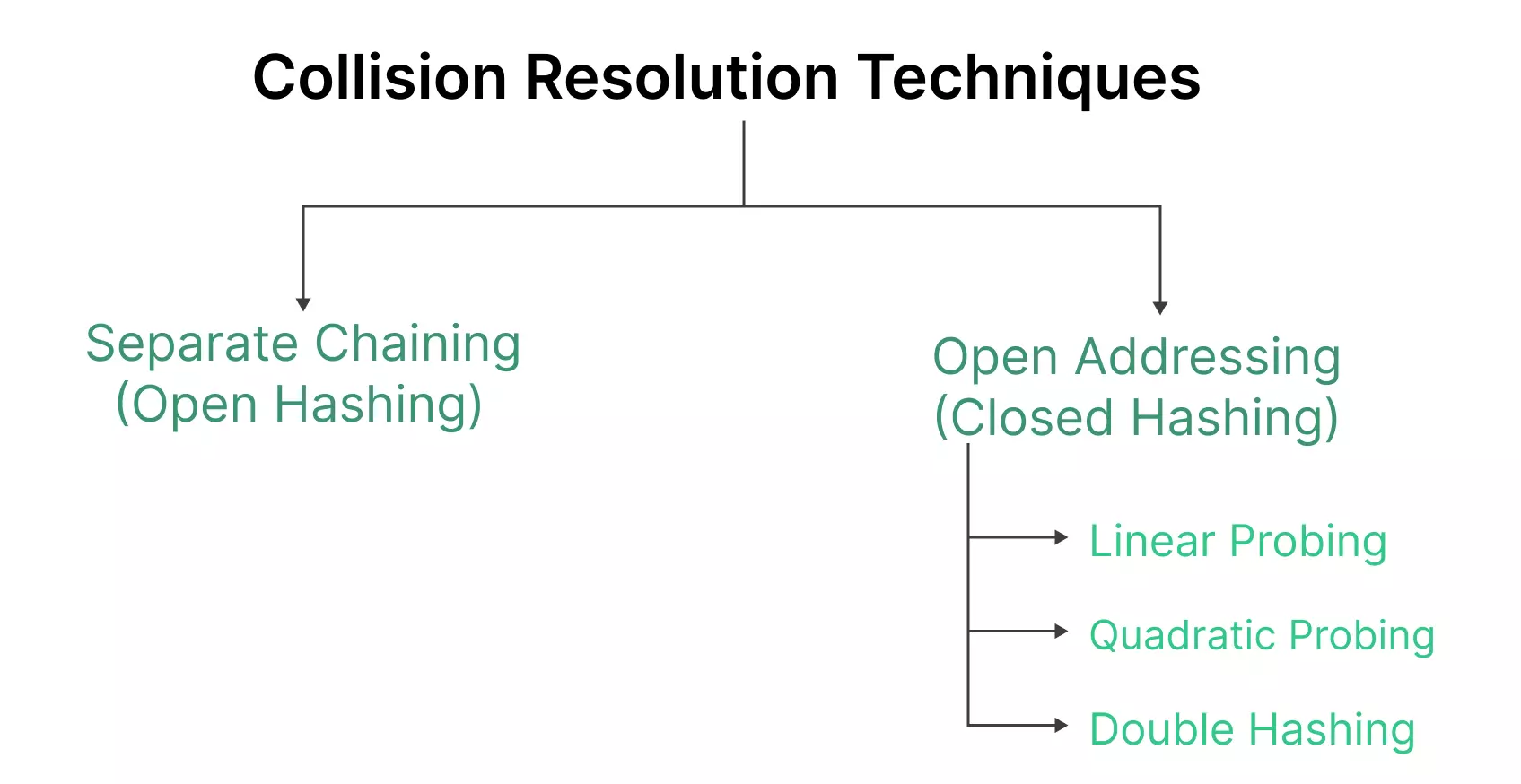Quadratic probing involves three main steps:

• Calculate the initial hash position for the key.
• If the position is occupied, apply the quadratic probing formula to find the next available slot.
• Repeat this process until an empty slot is found, and insert the data.
```New Position = (Initial Position + C1 * i + C2 * i^2) % Table Size
```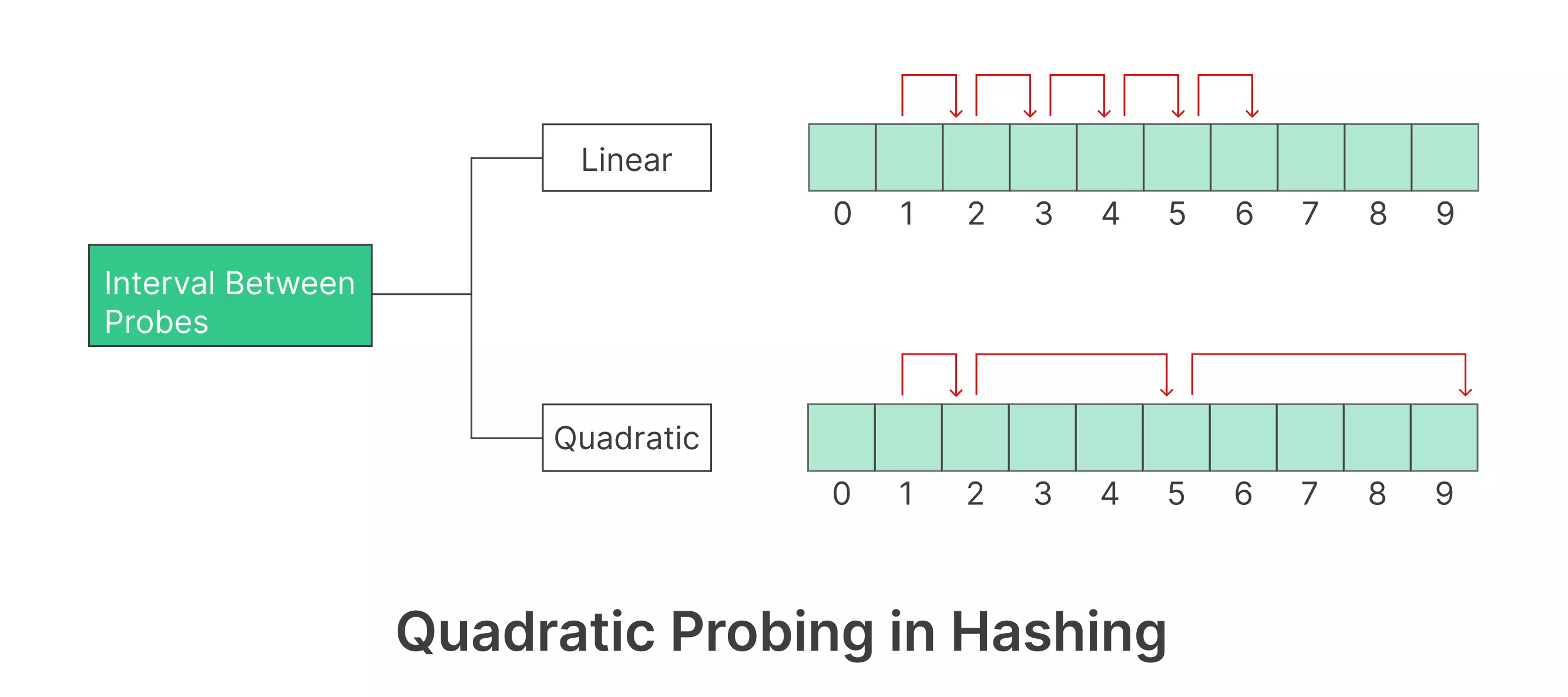## Example of Quadratic Probing in Hashing

Table size = 7 Insert = 15,22, 89 , Search & Delete = 85 , Hash Function –

Hash(x)=x%7 Collision Resolution Strategy f(i)= i^2

Step 1 – Create a table of size 7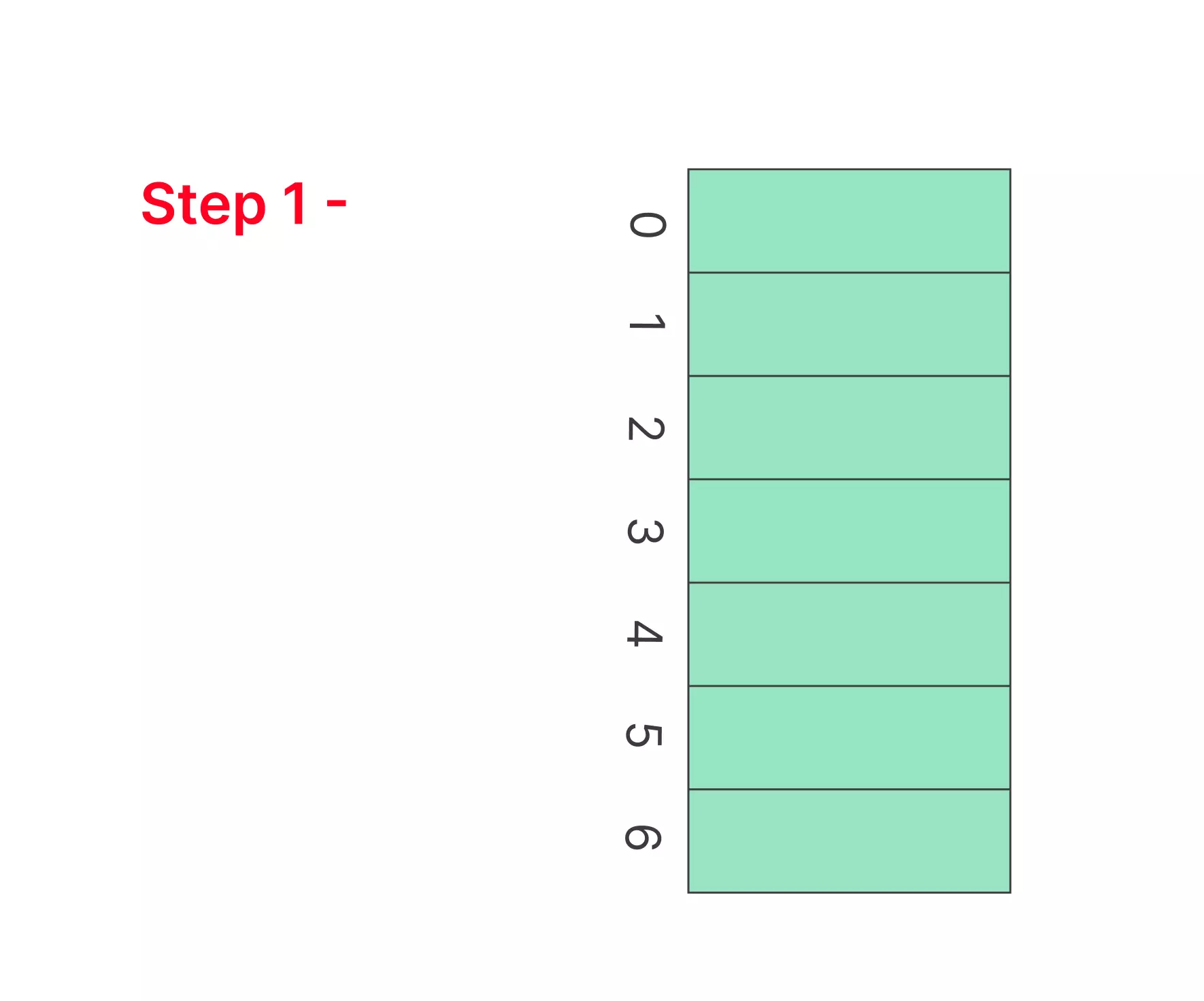Step 2 -Insert 15, 22

• hash(15) = 15%7 = 1 cell at index 1 is not occupied we can insert cell in index 1.
• hash(22) = 22%7 = 2 Again cell is not occupied so place cell in index 2.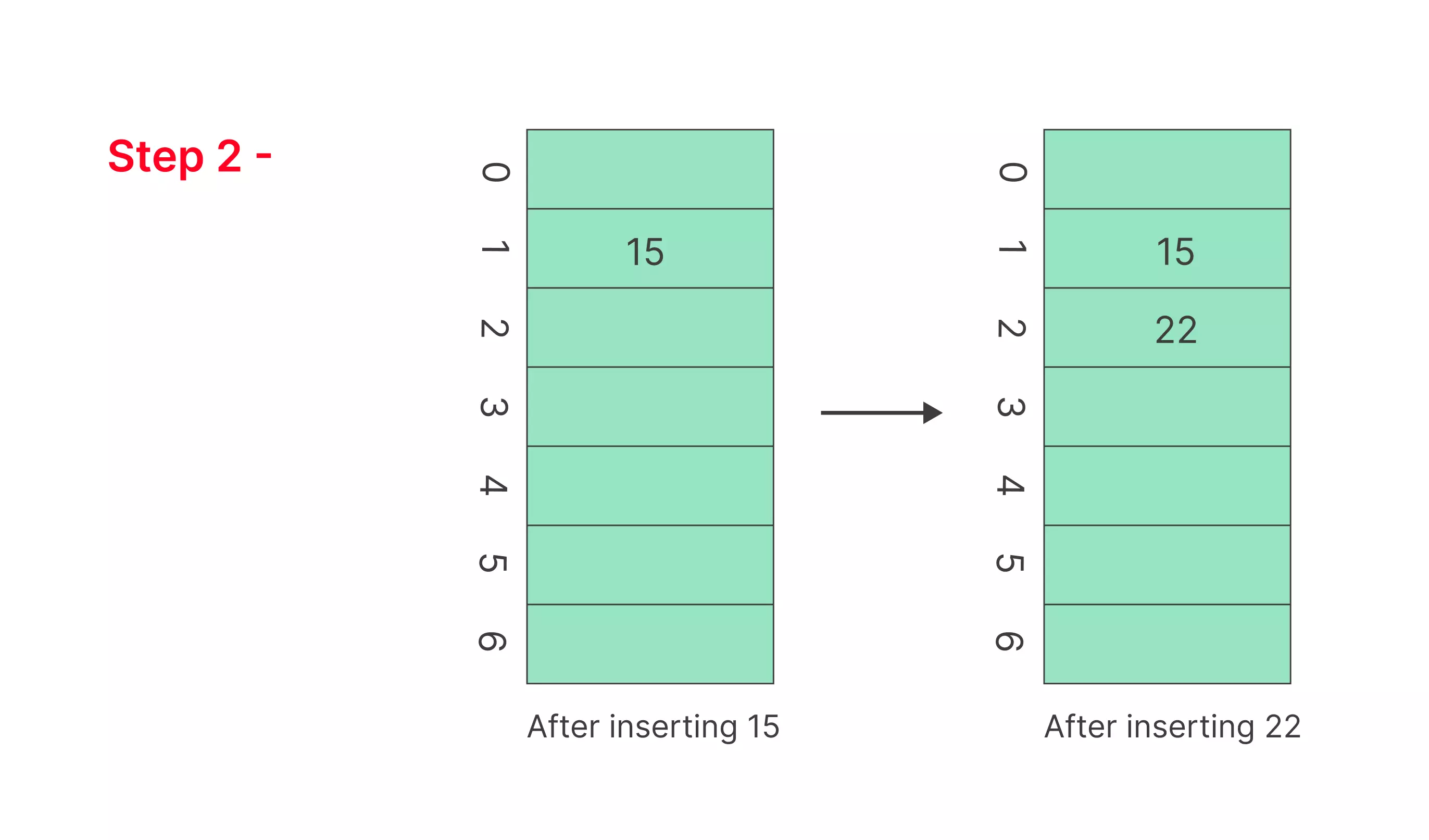Step 3 – Inserting 85

• hash(85) = 85%7 = 1 , Since cell 1 is already occupied So we will search for the equation :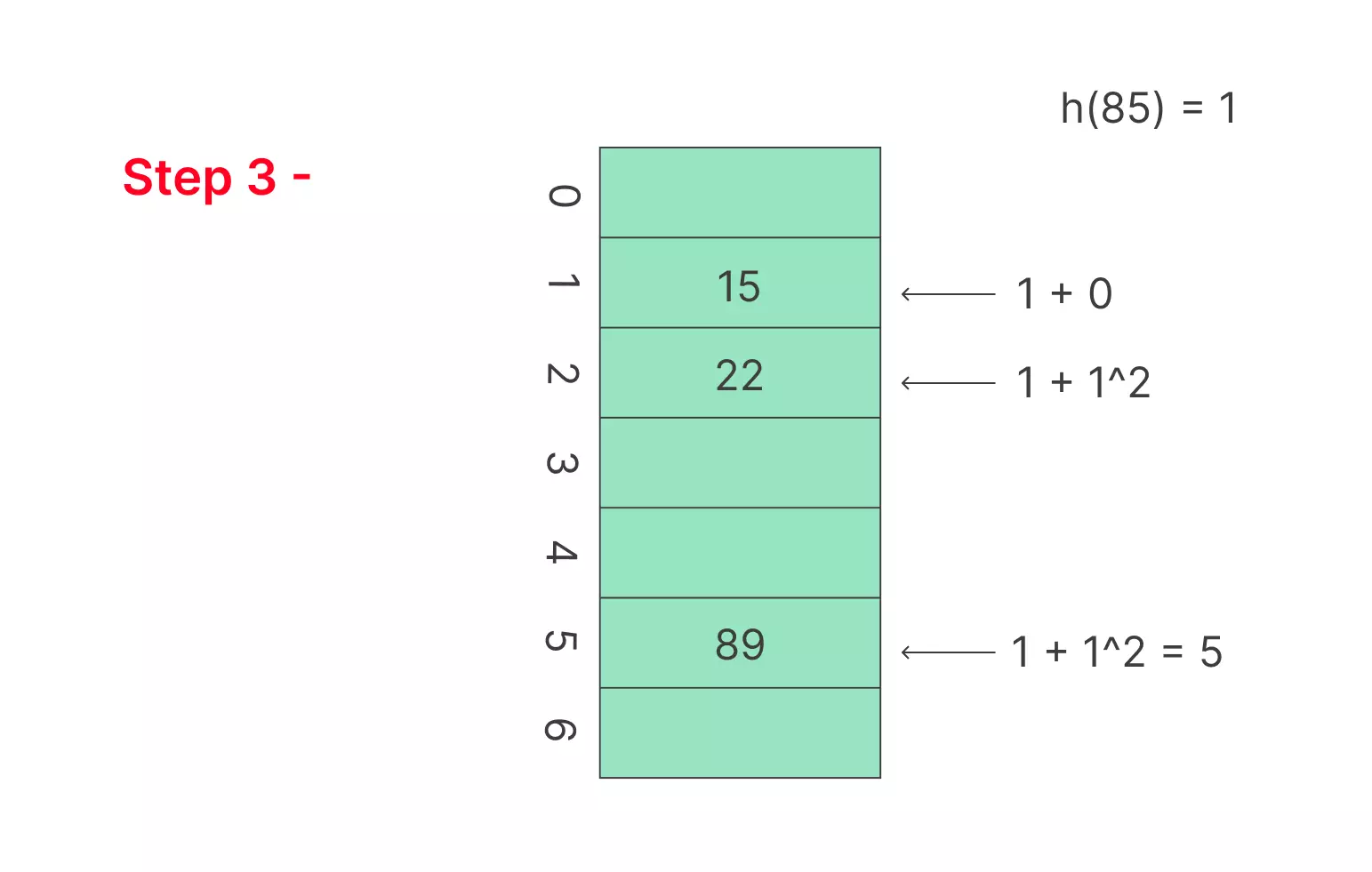## Implementing Quadratic Probing in Hashing

```# Define the hash table
table_size = 10
hash_table = [None] * table_size<
index = hash(key) % table_size
i = 0
while hash_table[index] is not None:
index = (index + i**2) % table_size
i += 1
hash_table[index] = key

# Example usage
```

## Comparing Quadratic Probing with Other Techniques

### Linear Probing vs. Quadratic Probing

While Linear Probing is straightforward, Quadratic Probing offers better performance due to its reduced clustering. However, Quadratic Probing may consume more memory.

### Quadratic Probing vs. Double Hashing

Double Hashing is even more efficient than Quadratic Probing but can be more complex to implement. Quadratic Probing strikes a balance between simplicity and performance.

## Applications of Quadratic Probing in Hashing

Quadratic probing is widely used in scenarios where quick data retrieval is crucial. Some common applications include:

### Conclusion

In Conclusion, , Quadratic Probing in Hashing emerges as a reliable technique to reduce clustering and enhance data retrieval efficiency. While it may not be the perfect solution for every scenario, it strikes a balance between simplicity and performance, making it a valuable tool in the world of data structures and algorithms.

### Related Banners

Get PrepInsta Prime & get Access to all 200+ courses offered by PrepInsta in One SubscriptionQuestion 1.

Is Quadratic Probing the best hashing technique?

Quadratic Probing is a good technique, but the best choice depends on the specific requirements of your application.Question 2.

The load factor is the ratio of the number of elements stored in the hash table to the table size. A low load factor is ideal for Quadratic Probing.Question 3.

Can Quadratic Probing handle hash table resizing efficiently?

Resizing a hash table using Quadratic Probing can be more challenging than other techniques due to the nature of the probing formula.

## Get over 200+ course One Subscription

Courses like AI/ML, Cloud Computing, Ethical Hacking, C, C++, Java, Python, DSA (All Languages), Competitive Coding (All Languages), TCS, Infosys, Wipro, Amazon, DBMS, SQL and others